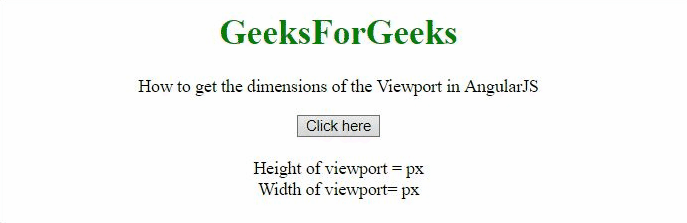# How to get the dimensions of the Viewport using AngularJS ?

In this article, we will see how to get the dimensions of the Viewport in AngularJS.

Approach:

• The approach is to use clientWidth and innerWidth property.
• The maximum of these values should be the width and height respectively.

Example 1: In this example, the height and width are calculated in pixels.

## HTML

 `  ` `<``html``> ` ` `  `<``head``> ` `    ``<``script` `src``= ` `"https://ajax.googleapis.com/ajax/libs/angularjs/1.2.13/angular.min.js"``> ` `    `` ` ` `  `    ``<``script``> ` `        ``var myApp = angular.module("app", []); ` `            ``myApp.controller("controller", function(\$scope) { ` `            ``\$scope.height = ''; ` `            ``\$scope.width = ''; ` `            ``\$scope.getVPDimnsn = function() {     ` `                ``\$scope.width =  ` `                    ``Math.max(document.documentElement.clientWidth, ` `                            ``window.innerWidth || 0) ` `                ``\$scope.height =  ` `                    ``Math.max(document.documentElement.clientHeight, ` `                            ``window.innerHeight || 0) ` `                ``}; ` `            ``}); ` `    `` ` `  ` ` `  `<``body` `style``=``"text-align:center;"``> ` `    ``<``h1` `style``=``"color:green;"``>   ` `        ``GeeksForGeeks   ` `    ``  ` ` `  `     `  `    ``<``p``> ` `        ``How to get the dimensions of the  ` `        ``Viewport in AngularJS ` `    `` ` ` `  `    ``<``div` `ng-app``=``"app"``>   ` `        ``<``div` `ng-controller``=``"controller"``> ` `            ``<``button` `ng-click``=``'getVPDimnsn()'` `> ` `                ``Click here ` `            `` ` `            ``<``br``><``br``> ` `            ``Height of viewport = {{height}}px ` `            ``<``br``> ` `            ``Width of viewport= {{width}}px ` `            ``<``br``> ` `        ``  ` `    `` ` `    ` `   `  ``

Output:Example 2: In this example, the height and width are calculated in centimeters.

## HTML

 `  ` `<``html``> ` ` `  `<``head``> ` `    ``<``script` `src``= ` `"https://ajax.googleapis.com/ajax/libs/angularjs/1.2.13/angular.min.js"``> ` `    `` ` ` `  `    ``<``script``> ` `        ``var myApp = angular.module("app", []); ` `            ``myApp.controller("controller", function(\$scope) { ` `            ``\$scope.height = ''; ` `            ``\$scope.width = ''; ` `            ``\$scope.getVPDimnsn = function() {     ` `                ``\$scope.width =  ` `                    ``Math.max(document.documentElement.clientWidth, ` `                            ``window.innerWidth || 0) ` `                ``\$scope.height =  ` `                    ``Math.max(document.documentElement.clientHeight, ` `                            ``window.innerHeight || 0) ` `                ``}; ` `            ``}); ` `    `` ` `  ` ` `  `<``body` `style``=``"text-align:center;"``> ` `    ``<``h1` `style``=``"color:green;"``>   ` `        ``GeeksForGeeks   ` `    ``  ` ` `  `     `  `<``p``> ` `        ``How to get the dimensions of the Viewport in AngularJS ` `    `` ` ` `  `    ``<``div` `ng-app``=``"app"``>   ` `        ``<``div` `ng-controller``=``"controller"``> ` `            ``<``button` `ng-click``=``'getVPDimnsn()'` `> ` `                ``Click here ` `            `` ` `            ``<``br``> ` `            ``<``br``> ` `            ``Height of viewport = {{height * 2.54 / 96}}cm ` `            ``<``br``> ` `            ``Width of viewport= {{width * 2.54 / 96}}cm ` `            ``<``br``> ` `        ``  ` `    `` ` `    ` `   `  ``

Output:My Personal Notes arrow_drop_upCheck out this Author's contributed articles.

If you like GeeksforGeeks and would like to contribute, you can also write an article using contribute.geeksforgeeks.org or mail your article to contribute@geeksforgeeks.org. See your article appearing on the GeeksforGeeks main page and help other Geeks.

Please Improve this article if you find anything incorrect by clicking on the "Improve Article" button below.

Article Tags :
Practice Tags :

Be the First to upvote.

Please write to us at contribute@geeksforgeeks.org to report any issue with the above content.